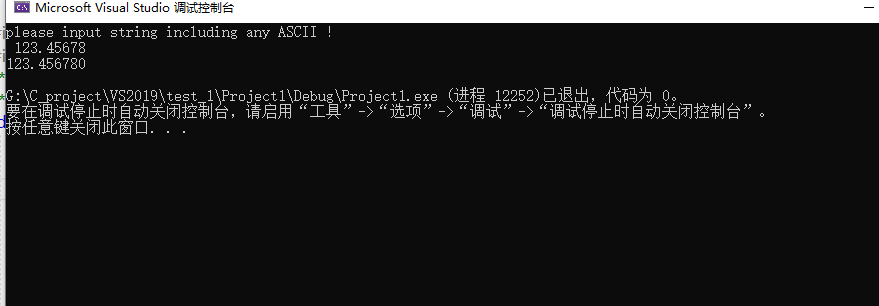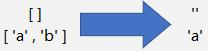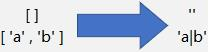• 字符串转浮点型数值函数–C语言 #include<stdio.h> #include<string.h> /*-----字符串转化为数字-----*/ /*-----只有当是数值型的字符串时，才可以完成字符串的转换。目前仅仅是完成了整数部分的转换，*...
字符串转浮点型数值函数–C语言
#include<stdio.h>
#include<string.h>
/*-----字符串转化为数字-----*/
/*-----只有当是数值型的字符串时，才可以完成字符串的转换。目前仅仅是完成了整数部分的转换，*/
double Uintnum(char* digit)
{
unsigned int num = 0;
double  num1 = 0;
while (*digit != '\0')
{
if (*digit >= '0' && *digit <= '9')
{
num = num * 10 + (*digit - '0');
digit++;
}
else
{
if (*digit == '.')
{
digit++;
break;
}
else
num = 0;
break;
}
}
while(*digit != '\0')
digit++;
digit = digit - 1;
while (*digit != '.')
{
if (*digit >= '0' && *digit <= '9')
{
num1 = num1*0.1 + (*digit - '0');
digit--;
}
else
{
num1 = 0;
break;
}
}
return(num + num1*0.1);
}
void main()
{
double data;
char a;
printf("please input string including any ASCII !  \n ");  //打印字符串

scanf_s ("%s", a,30);
/*----借助scanf_s输入字符串，这个函数包含三个参数，第一个是输入类型，第二个是变量名，第三个是输入字符串的上限*/
data = Uintnum(a);
printf("%lf \n", data);

}

运行结果如下所示：展开全文• 字符串转浮点型 取列表中的元素 并 转字符串 取列表第一个元素 取列表全部元素 应用场景 爬虫过程中，采集的数据常以str或float存入数据库； 遇到含小数点的文本，需要转换成浮点型 xpath()或re.findall()提取信息...
文章目录应用场景字符串转浮点型取列表中的元素 并 转字符串取列表第一个元素取列表全部元素
应用场景
爬虫过程中，采集的数据常以str或float存入数据库；

遇到含小数点的文本，需要转换成浮点型
xpath()或re.findall()提取信息返回列表，列表可能为空，不便存进数据库。

字符串转浮点型

float

a = float('-1.21')
print(a)


numpy

import numpy as np
ls = ['1.2', '3', '-0.5']
array = np.array(ls)
array = array.astype(float)
print(array)


pandas

import pandas as pd
d = {'num': ['-0.5', '1', '1.2']}
df = pd.DataFrame(d)
num = pd.to_numeric(df['num'])
print(num)

取列表中的元素 并 转字符串
取列表第一个元素ls1 = []
ls2 = [' a', 'b']
a = ls1.strip() if ls1 else ''
print(a)
a = ls2.strip() if ls2 else ''
print(a)

取列表全部元素ls1 = []
ls2 = ['\ta', '\nb\xa0']
a = '|'.join(ls1)
print(a)
a = '|'.join([i.strip() for i in ls2])
print(a)



展开全文• 很多面试题都会有字符串操作，网上一大堆都是用库函数实现，因此找不到一个纯c自己写的转换程序，所以post了这篇文章，记录自己的实现过程，以便日后再次复习
@纯C语言：字符串转浮点型（整型）
1.要求：变长字符串转实数：
解析：变长字符串经过 遍历 即可得出字符串的长度
非法字符的处理（即：0123456789. 之外的字符全为非法字符）
小数点前后数据处理
优点：本函数不需要考虑字符串前0 后0的情况，自动处理
注：可能因为字符串过长而被截取前n位有效位，后面自动截掉
#include "stdio.h"

double powff(float x, int n)  //  x的n次方
{
double result = 1;

while(n--)
{
result *= x;
}
return result;
}

double Str2Num(char *pStr)
{
double  Num = 0;
unsigned char BitBehidePoint = 0, leng=0, PointCount = 0;
while(pStr[leng] != '\0')
{
if ((pStr[leng] >= '0' && pStr[leng] <= '9') || pStr[leng] == '.')
{
if(pStr[leng] != '.' && !PointCount )
{
Num = Num*10 + (pStr[leng]-'0');
}
else if(PointCount )
{
BitBehidePoint ++;
Num += (pStr[leng] - '0') * powff(0.1, BitBehidePoint);
}
if(pStr[leng] == '.')
{
PointCount ++;
if(PointCount  >= 2)
{
printf("More point error \n");
return 0;
}
}
}
else
{
printf(" error sysm type \n");
return 0;
}
leng ++;
}
printf("the Strings’s leng is: %d  \n",leng);
return Num;
}

int main(void)
{
char strings = "123456.978654133";
printf("the result of String 2 Num is : %f\n", Str2Num(strings));
return 0;
}



展开全文c语言 数据结构 经验分享
• #include #include float mstrtof(char *num){ double n = 0, sign = 1, scale = 0; int subscale = 0, signsubscale = 1; if (*num == '-') { sign = -1, num++; /* Has sign? */ }
#include <stdio.h>
#include <math.h>

float mstrtof(char *num){

double n = 0, sign = 1, scale = 0;
int subscale = 0, signsubscale = 1;

if (*num == '-') {
sign = -1, num++;	/* Has sign? */
}

while (*num == '0') {
num++;
}

if (*num >= '1' && *num <= '9')	{
do {
n = (n * 10.0) + (*num++ - '0');
} while (*num >= '0' && *num <= '9');	/* Number? */
}

if (*num == '.' && num >= '0' && num <= '9') {
num++;
do {
n = (n * 10.0) + (*num++ -'0'), scale--;
} while (*num >= '0' && *num <= '9');
}	/* Fractional part? */

if (*num == 'e' || *num == 'E')	{	/* Exponent? */
num++;
if (*num == '+') {
num++;
} else if (*num == '-') {
signsubscale = -1, num++;		/* With sign? */
}

while (*num >= '0' && *num <= '9' ) {
subscale = (subscale * 10) + (*num++ - '0');	/* Number? */
}
}

n = sign * n * pow(10.0, (scale + subscale * signsubscale));	/* number = +/- number.fraction * 10^ +/- exponent */

return n;
}

int main(int argc, char *argv[])
{
char *temp = "12.34e-1";

printf("%f\n", mstrtof(temp));

return 0;
}


展开全文c语言 数据
• char – 字符型，占用1个字符的空间。一个float可转换为4个char表示。第一种方法，最简单的方法：unionfloat_type { floatf; chars; };注意数据存储的方向性（即大小端问题）。例如：在小端上： 浮点数3.750000...
• /**  * @brief: 从ptr开始扫描字符串，过滤到前面的空格，直到数字或者“+”、“-”号，  * 才开始转换。如果遇到非数字以及字符串结束符'\0'时，结束转换。... * @return: 转换后的浮点型数  */
• 现有问题： char *a = "180.01"; float f; f = atol(a); 此时f的值为180.00999 而我希望的值为180.01000 求高手解答！！！
• DIGITS={'0':0,'1':1,'2':2,'3':3,'4':4,'5':5,'6':6,'7':7,'8':8,'9':9} def str2float(s): s=s.split('.') if s==0: return 0+reduce(lambda x,y:x/10+y , map(lambda x:DIGITS[x],s[::-1]...
• '2.1' str3 = '三' str4 = '3.3.3.3' print(str1.isnumeric()) print(str2.isnumeric()) print(str3.isnumeric()) print(str4.isnumeric()) 结果： True False True False 那么如何识别浮点型数字的字符串呢？...
• C语言提供了几个标准库函数，可以将任意类型(整型、长整型、浮点型等)的数字转换为字符串。 1.int/float to string/array: C语言提供了几个标准库函数，可以将任意类型(整型、长整型、浮点型等)的数字转换为字符串，...
• java中String字符串转化为...// 转换为浮点型： // 使用Double或者Float的parseDouble或者parseFloat方法进行转换 // String s = "123.456 "; //要确保字符串为一个数值，否则会出异常 // double d = Double.parseDoubl
• 一个程序可以创建字符串并连接字符串，但不能截取子串，检查字符串的大小，检测字符串的内容。在Lua中操纵字符串的功能基本来自于string库。 字符串库中的一些函数是非常简单的： string.len(s) 返回字符串s的长度；...
• 原因：原来代码$price是带千分符的字串3,000.00，在和数量相乘时发生强制类型转换，转换为3，在js中去掉千分符，然后parseFloat,在html中去掉千分符，然后doubleFloat,不能转换为整型，会损失精确度 。 拖动进度条... • mysql 转换类型用两个函数：CONVERT（字段/需要转换的值, 类型） CAST（字段/需要转换的值 AS 类型） 因为之前设计，将某表金额字段类型设置为varchar 现在需要对此表根据用户，和表数据的类型进行汇总。...mysql 函数 CONVERT • 2 数值类型2.1 整型 十进制形式：普通整数 二进制形式：0b或0B开头的整数 八进制形式：0o或0O开头的整数 十六进制形式：0x或0X开头的整数2.2 浮点型 十进制形式：3.14、31.0、0.314等。 科学计数形式：3.14e2(3.14*... • ，该类的作用是将 一个字符串 转换成浮点型 (double )数值 。api java • python,字符串浮点型转整型问题ValueError: invalid literal for int() with base 10错误原因 ValueError: invalid literal for int() with base 10 复原错误： str_a = '1.5' int_a = int(str_a) 修正错误： # ...python • 1、字符串转int：parseInt() var perAmount =$("input[name=perAmount]").val();  var totalAmount = \$("input[name=totalAmount]").val();    if(totalAmount===""||parseInt(totalAmount)===0){js
•  转换为浮点型：  使用Double或者Float的parseDouble或者parseFloat方法进行转换 String s = "123.456 "; //要确保字符串为一个数值，否则会出异常 double d = Double.parseDouble(s); float f = Float....
• 1、字符串转化为整形、浮点类型String s = "100";//方法一int a = Integer.parseInt(String s);Long.parseLong(String s);Float.parseFloat(String s);Double.parseDouble(String s)//方法二int a = Integer.valueOf...
• 浮点型/整形转字符串转字符串 前言  有时我们在编写ESP8266程序的时候，库函数提供的sprintf()函数不能将浮点型数据转为字符串。或者在编写内存较小的单片机时，使用sprintf()等库函数会占用较大的代码空间，这时...c++
• 二进制数转字符串。给定一个介于0和1之间的实数（如0.72），类型为double，打印它的二进制表达式。 如果该数字不在0和1之间，或者无法精确地用32位以内的二进制表示，则打印“ERROR”。 示例1: 输入：0.625 输出：...
• Python字符串、整数、和浮点型数相互转换实例前言序锦在编程中，经常要用到字符串的相互转换，现在在这里记录一下Python里面的字符串和整数以及浮点型数之间是如何进行相互转换的。int(str)函数将符合整数的规定的...
• 最近在做接口，使用的是jemter工具，在使用jemter工具中，基础的和高级的，在贺满的博客中可以查看到，https://www.cnblogs.com/puresoul/p/5092628.html，我在使用操作上补充一些自己遇到的一些坑 ...字符串转int...
• pycharm中字符串内容为浮点型时无法直接转换为整型。 如图： 应当将a转化为浮点型再转化为整型。 反之字符串内容为整型，可直接转为浮点型 本文为初学者学习笔记，如有错误望大佬指出，内容具体原因还望大佬指点，...python...